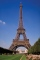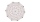# An equilateral triangle

The perimeter of an equilateral triangle is 33cm. How long is each side?

Result

a =  11 cm

#### Solution:Leave us a comment of this math problem and its solution (i.e. if it is still somewhat unclear...):Be the first to comment!## Next similar math problems:

1. Axial sectionAxial section of the cone is an equilateral triangle with area 208 dm2. Calculate the volume of the cone.
2. Trapezoid MOThe rectangular trapezoid ABCD with right angle at point B, |AC| = 12, |CD| = 8, diagonals are perpendicular to each other. Calculate the perimeter and area of ​​the trapezoid.
3. GardenArea of a square garden is 6/4 of triangle garden with sides 56 m, 35 m, and 35 m. How many meters of fencing need to fence a square garden?
4. Area of a rectangleCalculate the area of a rectangle with a diagonal of u = 12.5cm and a width of b = 3.5cm. Use the Pythagorean theorem.
5. RectangleThe rectangle is 21 cm long and 38 cm wide. Determine the radius of the circle circumscribing rectangle.
6. Angles of elevationFrom points A and B on level ground, the angles of elevation of the top of a building are 25° and 37° respectively. If |AB| = 57m, calculate, to the nearest meter, the distances of the top of the building from A and B if they are both on the same side of t
7. Tetrahedral pyramidWhat is the surface of a regular tetrahedral (four-sided) pyramid if the base edge a=16 and height v=16?
8. Faces diagonalsIf the diagonals of a cuboid are x, y, and z (wall diagonals or three faces) respectively than find the volume of a cuboid. Solve for x=1.2, y=1.7, z=1.45
9. Two chordsCalculate the length of chord AB and perpendicular chord BC to circle if AB is 4 cm from the center of the circle and BC 8 cm from the center of the circle.
10. The Eiffel TowerThe top of the Eiffel Tower is seen from a distance of 600 meters at an angle of 30 degrees. Find the tower height.
11. Depth anglesAt the top of the mountain stands a castle, which has a tower 30 meters high. We see the crossroad in the valley from the top of the tower and heel at depth angles of 32° 50 'and 30° 10'. How high is the top of the mountain above the crossroad
12. Right ΔA right triangle has the length of one leg 7 cm and length of the hypotenuse 25 cm. Calculate the height of the triangle.
13. Wall and body diagonalsCalculate the lengths of the wall and body diagonals of the cuboid with edge dimensions of 0.5 m, 1 m, and 2 mThe ladder has a length of 3 m and is leaning against the wall, and its inclination to the wall is 45°. How high does it reach?The volume of a right circular cone is 5 liters. Calculate the volume of the two parts into which the cone is divided by a plane parallel to the base, one-third of the way down from the vertex to the base.What is the length d of the chord circle of diameter 50 dm, if the distance from the center circle is 21 dm?In right triangle is: ? Determine the value of s and c: ? ?